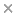BaseSpace
Correlation Engine 2.0Search sequence regions

filter terms:

The power of a chi-square test, and thus the required sample size, are a function of the noncentrality parameter that can be obtained as the limiting expectation of the test statistic under an alternative hypothesis specification. Herein, we apply this principle to derive simple expressions for two tests that are commonly applied to discrete ordinal data. The Wilcoxon rank sum test for the equality of distributions in two groups is algebraically equivalent to the Mann-Whitney test. The Kruskal-Wallis test applies to multiple groups. These tests are equivalent to a Cochran-Mantel-Haenszel mean score test using rank scores for a set of C-discrete categories. Although various authors have assessed the power function of the Wilcoxon and Mann-Whitney tests, herein it is shown that the power of these tests with discrete observations, that is, with tied ranks, is readily provided by the power function of the corresponding Cochran-Mantel-Haenszel mean scores test for two and R > 2 groups. These expressions yield results virtually identical to those derived previously for rank scores and also apply to other score functions. The Cochran-Armitage test for trend assesses whether there is an monotonically increasing or decreasing trend in the proportions with a positive outcome or response over the C-ordered categories of an ordinal independent variable, for example, dose. Herein, it is shown that the power of the test is a function of the slope of the response probabilities over the ordinal scores assigned to the groups that yields simple expressions for the power of the test. Copyright © 2011 John Wiley & Sons, Ltd.

Citation

John M Lachin. Power and sample size evaluation for the Cochran-Mantel-Haenszel mean score (Wilcoxon rank sum) test and the Cochran-Armitage test for trend. Statistics in medicine. 2011 Nov 10;30(25):3057-66

PMID: 22006667

View Full Text